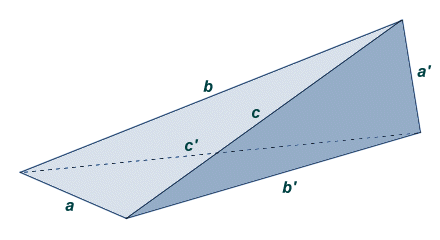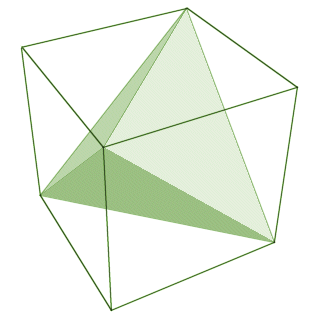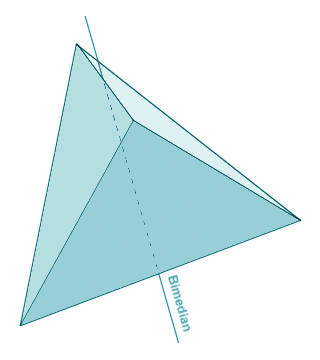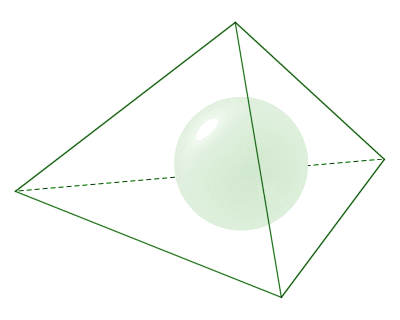# The Tetrahedron

The tetrahedron is a special case of the pyramid in the sense that it is a triangular pyramid (i.e. all of the faces are triangles, including the base polygon). The general characteristics of pyramids are dealt with in the page entitled "Pyramids". On this page, we will be looking specifically at the properties of tetrahedra. Like all pyramids, the tetrahedron is a polyhedron (i.e. a three-dimensional geometrical shape with flat faces and straight edges). It has four (4) faces (the word tetra has its origins in the Greek language, and means four), six (6) edges, and four (4) vertices. What distinguishes the tetrahedron from other pyramids is that all of its faces are triangles. As you can see from the illustration below, the tetrahedron has a triangular base (any one of the tetrahedron's four faces can be designated to be the base), and three triangular sides that connect the base to the apex. Each vertex of the tetrahedron is shared by three of its triangular faces.All of the faces of a tetrahedron are triangles, including the base

The tetrahedron is the only polyhedron that has four faces. It is also the only simple polyhedron that has no polyhedron diagonals (i.e. no face diagonals or space diagonals). An isosceles tetrahedron is a special case of the general tetrahedron for which all four of the triangular faces are congruent. A regular tetrahedron is a special case of both the general tetrahedron and the isosceles tetrahedron for which all four triangular faces are not only congruent, but are also equilateral triangles, i.e. triangles for which all three sides are the same length, and all internal angles are sixty degrees (60°). The illustration below shows an isosceles tetrahedron.The faces of an isosceles tetrahedron are congruent triangles

In an isosceles tetrahedron, each pair of opposite edges are congruent (i.e. equal in length). With reference to the above illustration, therefore, we have:

a  =  a'

b  =  b'

c  =  c'

From this, it should be relatively easy to see that the four faces of the isosceles tetrahedron are congruent, since each of the four triangular faces is composed of one of the following combinations of sides:

a, b, c

a, b', c'

a', b', c

a', b, c'

If you look closely at the illustration, you should be able to see that the three face angles that meet at each vertex of the tetrahedron consist of the angle between sides a (or a') and b (or b'), plus the angle between sides a (or a') and c (or c'), plus the angle between sides b (or b') and c (or c'). Since each vertex is thus effectively formed by three different internal angles from triangles that are congruent, and since the three internal angles of a triangle always add up to one hundred and eighty degrees (180°), then the face angles at each vertex of an isosceles tetrahedron must also sum to one hundred and eighty degrees.

As we have already mentioned, the regular tetrahedron is a special case of the isosceles tetrahedron for which all four faces are congruent equilateral triangles. The regular tetrahedron is one of the five platonic solids (a platonic solid is a regular convex polyhedron with all faces being regular congruent polygons, and the same number of faces meeting at each vertex). The regular tetrahedron is the only regular polyhedron with no parallel faces, and has a number of other characteristics that follow from the fact that all of its faces are congruent equilateral triangles:

• all edges are of the same length
• all internal angles have a magnitude of 60°
• the four vertices are equidistant from one another
• opposite edges are perpendicular (at right-angles) to one another
• four axes of symmetry pass through each vertex and the centroid of its opposite face
• three axes of symmetry pass through the midpoints of opposite edges
• the dihedral angle between any two faces has a magnitude of (circa) 70.53°
• the angle between an edge and a face has a magnitude of (circa) 54.74°

A regular tetrahedron can be formed using six of the face diagonals of a cube, as shown below. The vertices of the tetrahedron are coincident with four of the cube's vertices. The figure clearly illustrates some of the characteristics of a regular tetrahedron listed above. For example, since each edge of the tetrahedron is also one of the cube's face diagonals, the edges of the tetrahedron must be equal in length. Furthermore, since each of the tetrahedron's vertices is connected to every other vertex by an edge that is also one of the cube's face diagonals, they must be equidistant. Finally, each pair of opposite edges for the tetrahedron consists of a pair of alternate face diagonals belonging to opposite faces of the cube. They must therefore be perpendicular to one another.A regular tetrahedron shares four vertices with a cube

Since all of the faces of a regular tetrahedron are equilateral triangles, all of the internal angles of the tetrahedron will be sixty degrees (60°), and the sum of the face angles for the three faces meeting at any vertex will be one hundred and eighty degrees (180°). The axes of symmetry are of particular significance when we are dealing with regular tetrahedra. Remember that there is an axis of symmetry that connects each vertex of the regular tetrahedron with the centroid of its opposite face (see the illustration below). By definition, the line passing through any vertex of a tetrahedron and the centroid of the face opposite that vertex is called a median. A tetrahedron thus has four (4) medians. For a regular tetrahedron, each median is coincident with one of its axes of symmetry.For a regular tetrahedron, each median is coincident with an axis of symmetry

A similar situation occurs when we consider the axis of symmetry that connects the mid-point of two opposite edges of a regular tetrahedron (see the illustration below). By definition, the line passing through the mid-points of two opposite edges for any tetrahedron is called a bimedian. A tetrahedron will therefore have three (3) bimedians, because it has three pairs of opposite edges. Again, for a regular tetrahedron, each bimedian is coincident with one of its axes of symmetry.For a regular tetrahedron, each bimedian is coincident with an axis of symmetry

The four medians and three bimedians of a tetrahedron (and therefore by definition the seven axes of symmetry for a regular tetrahedron) all intersect at a single point called the centroid (or geometric centre) of the tetrahedron. If the tetrahedron were a solid object of uniform density, the centroid would be that object's centre of mass.

We mentioned above that a regular tetrahedron may share four vertices with a cube, but there is another (non-regular) type of tetrahedron called a trirectangular tetrahedron for which all three face angles at one of its vertices are right-angles. You can visualize what this tetrahedron might look like by imagining what you would get if you sliced off one corner of a cube. The illustration below attempts to clarify this idea.A trirectangular tetrahedron has a single vertex at which all three face angles are right-angles

For all tetrahedra, there exists a sphere called the circumsphere which completely encloses the tetrahedron. The tetrahedron's vertices all lie on the surface of its circumsphere. The point at the centre of the circumsphere is called the circumcentre.All of the tetrahedron's vertices lie on the surface of its circumsphere

Note that for a regular tetrahedron with an edge length of a, the radius of the circumsphere is given by the following formula:

 Radius of circumsphere  =  √ 3 a 8

There also exists for all tetrahedra a sphere known as the insphere that is completely enclosed by the tetrahedron. The insphere is tangent to each of the tetrahedron's four faces, i.e. the insphere touches the surface of each face at a single point. The point at the centre of the insphere is called the incentre.The tetrahedron's insphere is tangent to all of its faces

Note that for a regular tetrahedron with an edge length of a, the radius of the insphere (which is exactly one third of the radius of the circumsphere) is given by the following formula:

 Radius of insphere  = a √24

Note that for any isosceles tetrahedron (of which the regular tetrahedron is a special case) the circumsphere and insphere are concentric (i.e. they share a common centre), which means that the circumcentre and incentre will be coincident.

Getting down to more mundane issues, the volume and surface area of a tetrahedron can be found using the same methods as for any other pyramid. The volume V of a tetrahedron is calculated as one third of its base area B (regardless of which of the four faces is taken to be the base) multiplied by its height h. We can formalise this relationship as:

 V  = 1 Bh 3

Note that for a regular tetrahedron with an edge length of a, the volume can be found using the following alternative formula:

 V  = √2 a3 12

Finding the surface area of a (non-regular) tetrahedron is usually a little more complicated, since the four triangular faces may all have different areas. If this is the case, the area of each face must be found separately, and the values added together to get the total surface area. If the tetrahedron is isosceles, life is somewhat simpler because the four faces will be congruent, and will therefore all have the same area. Note that for a regular tetrahedron with an edge length of a, the total surface area A can be found using the following formula:

A  =  √3a2

The tetrahedron is of interest to mathematicians for its own sake, thanks to its fairly unique characteristics among polyhedra. It is also studied by scientists, engineers, and practitioners in other areas for a number of reasons. It is of interest in the field of chemistry, for example, because the molecules of various chemical compounds (water and methane, for example) have a tetrahedral structure. In the field of electronics, the tetrahedron is of significance because silicon crystals have a tetrahedral structure. This is due to the fact that silicon atoms have a valence of four (the valence of an atom indicates the number of chemical bonds it can form with other atoms). Silicon is the semiconductor material most commonly used in the production of electronic circuit boards and components, so its crystalline structure has naturally been the subject of extensive study.